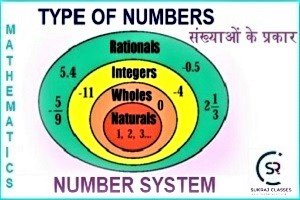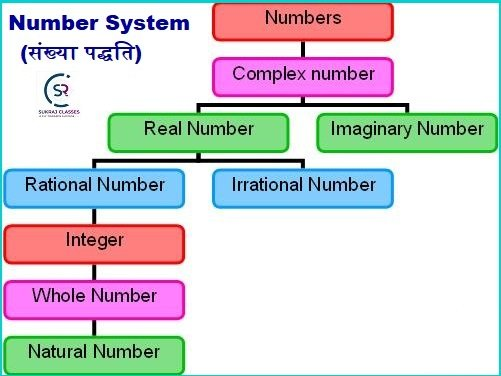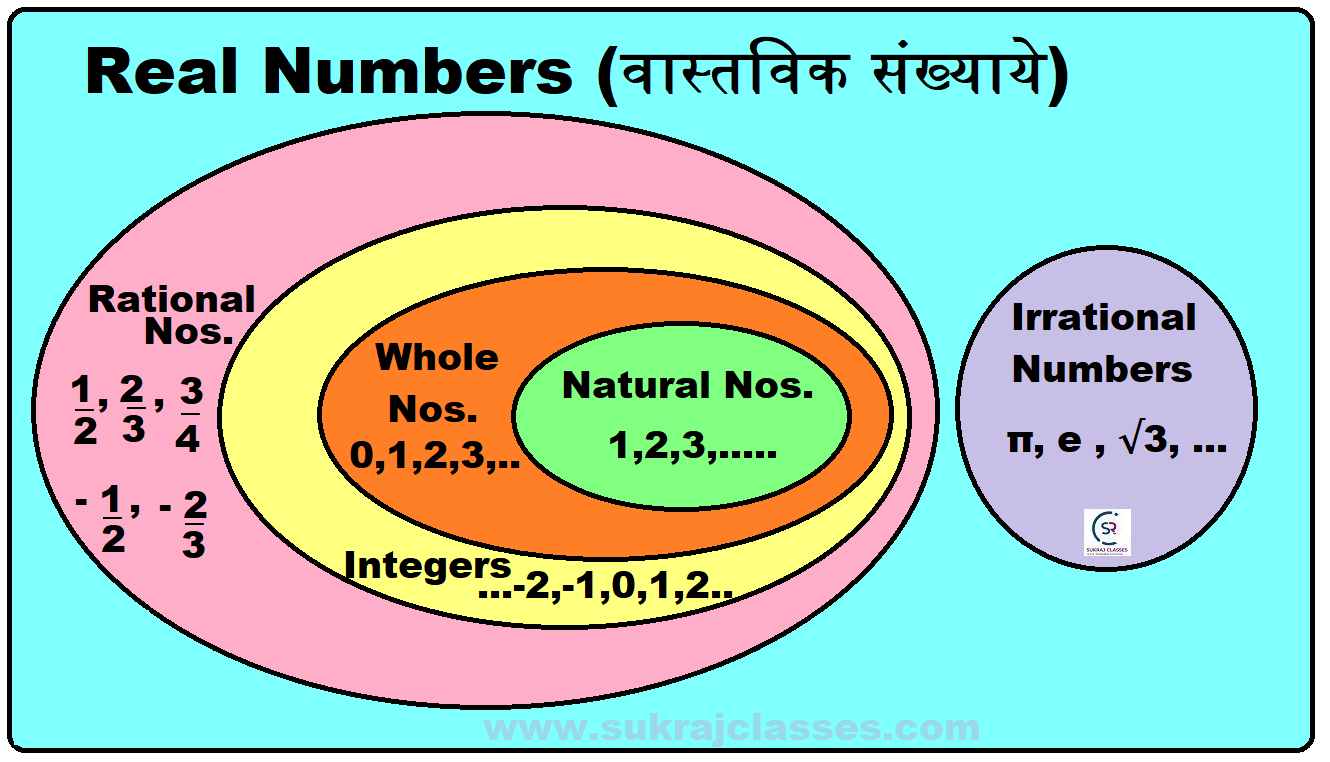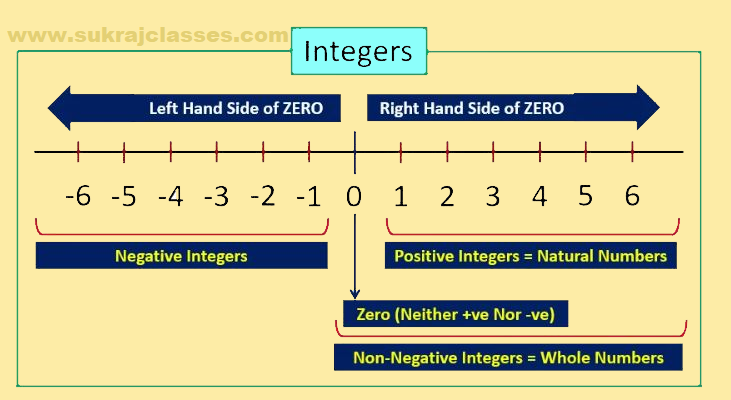# Types of Numbers – Number System

Mathematics topic – “Types of Numbers – Number System “, is important for all competitive exams like CET- Common eligibility Test,  SSC CGL, SSC CHSL, RRB NTPC, UPSC and other State PCS Exams. Mathematics is the most reliable and marks obtaining subject in competitive exams.

Number System

In number system, first we need to understand the types of numbers so that we can use at our requirement in Mathematics.### Types of Numbers

Natural numbers (N) – प्राकृतिक संख्या

Natural Numbers = N = {1, 2, 3,……}

• All the counting numbers are known as natural numbers or Numbers which start from 1 to infinity (No negative numbers and No fractions).
• The natural numbers are also called positive integers and start with 1.
• The natural numbers are denoted by N. The number of natural numbers are infinite.
• The natural numbers were the first number system developed and primarily used in counting.

For Example: – 1, 2, 3, 4………..Whole Numbers (W) – पूर्ण संख्या

Whole Numbers = N = {0, 1, 2, 3,…}

• Numbers which start from 0 to infinity (with no fractional part and no decimals).
• इसे ऐसे समझें :- In natural numbers, the numbers start with 1. There is no number used for nothing, means zero (0). Thus, when zero (0) is included in the set of natural numbers, then it is known as whole numbers. Hence, the whole numbers start with zero (0).
• The set of whole numbers are denoted by W and it is also called as Non-Negative Integers.
• For Example: – 0, 1, 2, 3, 4…….
• On comparing the sets of natural numbers N = {1, 2, 3…} and whole numbers W = {0, 1, 2, 3…}, we get that the natural numbers set is the subset of whole numbers. Thus, N ⊂ W.

Integers (Z) – पूर्णांक

Integers = Z = {…, -3, -2, -1, 0, 1, 2, 3 …}

• Positive and negative numbers including Zero but no fractional part (no decimals) are called integers.
• Example of Integers = Z = {…, -3, -2, -1, 0, 1, 2, 3, …}
• इसे ऐसे समझें :- Integers are the set of natural numbers N = {1, 2, 3, ,…}, zero (0) and the negative of the natural numbers {-1, -2, -3, ,…}. Thus, the integers are {…,-3, -2, -1, 0, 1, 2, 3…}.
• The set of the integers are denoted by Z (starting letter of German word ZAHLEN which means integers).
• On comparing the sets of natural numbers N = {1, 2, 3…..}, whole numbers W = {0, 1, 2, 3…} and the integers Z = {…, -3, -2, -1, 0, 1, 2, 3…}, we get N ⊂ W ⊂ Z.What is Zero (0)

Zero (0) is neither positive nor negative and it is located in between positive and negative integers on the number line.

Positive Integers

The set of positive integers is the natural numbers N = {1, 2, 3…} which is located on the right hand side of zero on the number line.

Negative Integers

• The set of negative integers is the negative of the natural numbers {-1, -2, -3…} which is located on the left hand side of zero on the number line.
• The negative integers are less than zero.

Non-Negative Integers

The set of non-negative integers is the whole numbers Z = {0, 1, 2, 3…}.

Rational Numbers (Q) – परिमेय संख्याएं

Rational Number (Q) = {x = p/q. where p and q ∈ Z and q ≠ 0}

• Numbers that can be written in the form of p/q where p and q are INTEGERS and q ≠ 0, are known as rational numbers.
• Either terminating or non-terminating recurring type.
• The set of the rational numbers are denoted by Q (starting letter of quotient).
• For Example: – (Q) = 2/3, 4/5, 22/7 etc.
• Each integers can be written in the form of p/q. For example: 8 = 8/1 or -2 = -2/1. Thus, each integer is a rational numbers.
• On comparing the sets of natural numbers N = {1, 2, 3…}, whole numbers W = {0, 1, 2, 3…} and the rational numbers Q = {…, -17/5, -12/7, 0, 5/3, 8…}, we get N Q

Irrational Numbersअपरिमेय संख्याएं

• Numbers which cannot be expressed in p/q form, where p and q are integer and q≠ 0, are known as irrational numbers.
• These are Non-terminating and non-repeating type.
• For example: – √2, √3, and pi (π) as π= 3.1415926535897932384626433832795… etc.

Real Numbers (R) – वास्तविक संख्याएँ

• The set of all rational and irrational numbers are known as real numbers.
• All Numbers on number line are real numbers.
• It can be denoted by R.
• It contains rational and irrational numbers both.
• For example: 1, 1/5, -1.25, 1.333, -25.3 18.25487… etc.
• The square of a real numbers is always positive.

x² ≥ 0 ⇒ x is REAL Number

Complex Numbers – मिश्र संख्या:

• Complex numbers are the numbers that are expressed in the form of a+ib. where, a and b are real numbers and ‘i’ is an imaginary number called “iota”.
• The value of i = (√-1).
• For example:-  2+3i is a complex number, where 2 is a Real number and 3i is an imaginary number.

Imaginary Numbers – काल्पनिक संख्याए:

A Number whose square is negative is known as imaginary number.

• For example: 2i, 3i, -6i etc where i=iota in Complex Number
• The imaginary numbers are used in complex number, which are in the form of a+ib.

x² < 0 ⇒ x is IMAGINARY Number

Prime Numbers – अभाज्य संख्याये:

• A number that can be divided exactly only by itself and 1, is called Prime Number
• 1 is neither prime number nor composite number.
• For example: – 2, 3, 7, 17, 41 etc.

Co-prime numbers – सह-अभाज्य संख्याये:

Those rational numbers which do not have any common factors other than 1, are called co-prime numbers.

For Example: 1/3, 5/6 etc.

Important points:

• There are infinite rational numbers between any two rational numbers.
• In between any two real numbers there are infinite real numbers.

To find out rational numbers between any two given rational numbers, there is mid value method

Let’s assume two given numbers are A and B, Then   (A+B)/2

Add to given numbers A and B and divide them by 2, we will get mid value in between two given numbers.

Composite Numbers समग्र संख्या:

• Composite numbers can be defined as the whole numbers that have more than two factors.
• Whole numbers that are not prime are composite numbers, because they are divisible by more than two numbers.
• 1 is neither prime number nor composite number.
• For Example: – 4 is Composite number = (2×2),    6 can be made by 2×3 so is NOT a prime number, it is a composite number

Whereas 2 is Prime, 3 is Prime, 4 is Composite (=2×2), 5 is Prime, and so on…

Perfect Numbers:

• A positive integer that is equal to the sum of its proper divisors, is known as the Perfect Number.
• The smallest perfect number is 6, which is the sum of 1, 2, and 3. Other perfect numbers are 28, 496, and 8128.

Even Numbers (सम संख्या) and Odd Numbers (विषम संख्या)

A number which is divisible by 2 and generates a remainder of 0 is called an even number.

• Any number that can be divided by two to create another whole number is even.
• For Example: – 2, 4, 6, 8 …..

An odd number is a number which is not divisible by 2.

• The remainder in the case of an odd number is always “1”.
• For Example: – 3, 5, 7, 9……

Note: zero (0) is an even number.

Fraction or Equivalent Rational Numbers:

• Those rational numbers have a common factor are called equivalent rational numbers. For example: 4/6, 25/50 etc.
• Numbers that can be written in the form of p/q, where p and q are WHOLE numbers and q ≠ 0, are called as fraction. For example: 5/3, 2/5, 1/2, 2/9 etc.
• In a fraction p/q (q ≠ 0), the number (p) written over the line known as NUMERATOR and the number (q) written below the line is known as DENOMINATOR is called as fraction.

Thus, by the definitions of rational numbers and fractions, we can say that:

RATIONAL NUMBER can be positive or negative, but FRACTION is always positive.

Decimals – (दशमलव संख्याए):

Decimal Number = Whole Part . Fractional Part

• A number whose whole part and the fractional part are separated by decimal point, is known as Decimal Number.
• For example: 2.7, 0.25, 3.6543 etc.
• In decimal number, as we move from the left to right, the place of the digits gets divided by 10.

Types of Decimals: – Terminating and Non-terminating Decimals.

Terminating Decimals

In the conversion of a fraction (p/q) into a decimal, when we get a remainder zero (0) in the division of the numerator p by the denominator q, then the decimal is terminated after some decimal places. This types of decimal is known as the terminating decimals.

For example: 17/5 = 3.4, 24/3 = 8.0, 1/80 = 0.0125 etc.

Non-Terminating Decimals

In the conversion of a fraction (p/q) into a decimal, when we don’t get a remainder zero (0) at any step in the division of the numerator p by the denominator q, then the decimal is NOT terminated after some decimal places. This types of decimal is known as the non-terminating decimals.

For example: 1/3 = 0.333…, 9/7 = 1.2563… etc.

Non-terminating decimals are also two types

1. Recurring decimals
2. Non-recurring decimals.

Recurring Decimals

• When we have a repeating digits after decimal point in the non-terminating, then it is known as recurring decimals.
• For example: 1/3 = 0.333…, 1/6 = 0.1666… 1.232323…., 1.785785785…etc.

Terminating and Recurring Decimals are RATIONAL Numbers

Non-Recurring Decimals

• When we have NO repeating digits after decimal point in the non-terminating, it is known as recurring decimals.
• For example: 1.2658743…, 1.02528954…, 1.10100100010000… etc.

Non-Recurring Decimals are IRRATIONAL Numbers

Types of Numbers – Number System

For :

If you like and think that Topic of Mathematics for competitive Exams – “Types of Numbers – Number System ” was helpful for you, Please comment us. Your comments/suggestions would be greatly appreciated. Thank you to be here. Regards – Team SukRaj Classes.